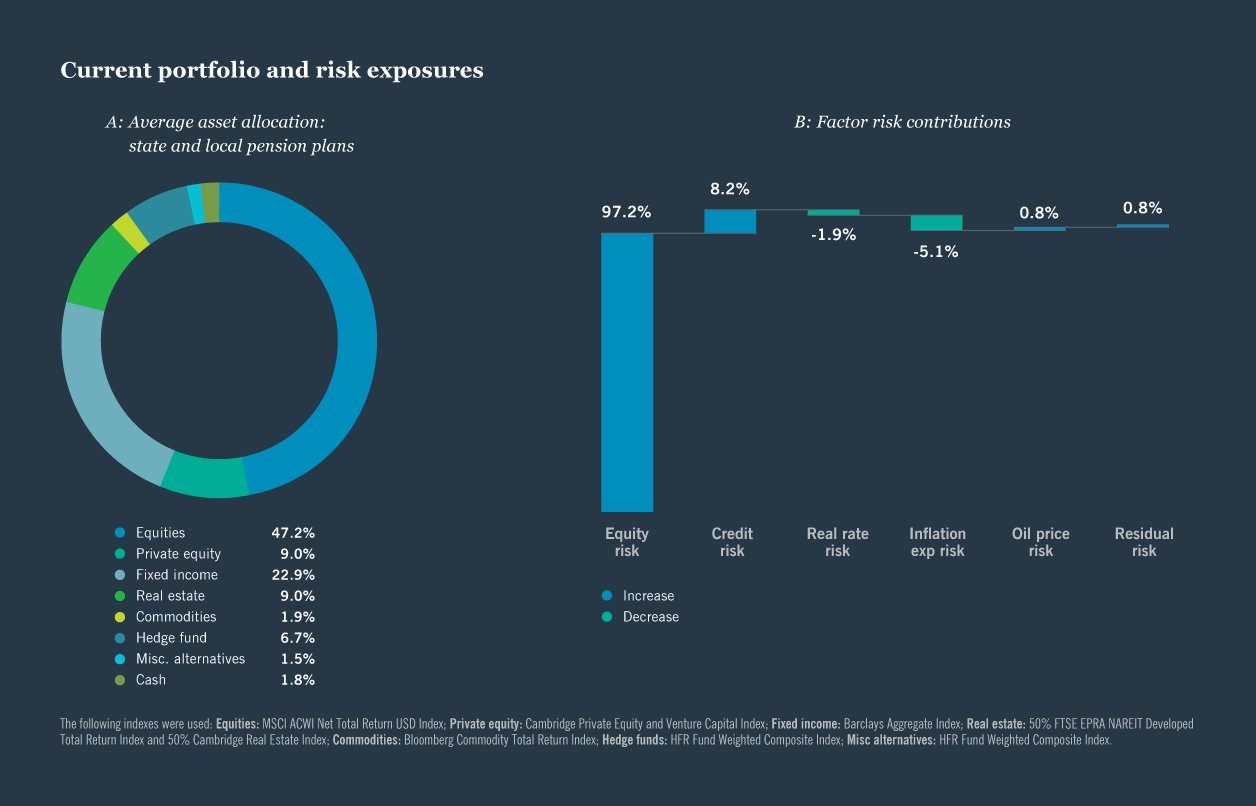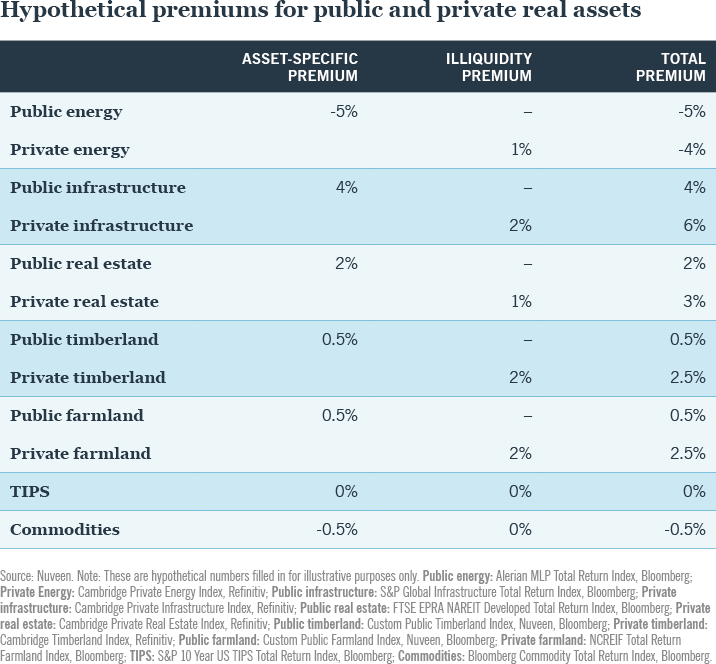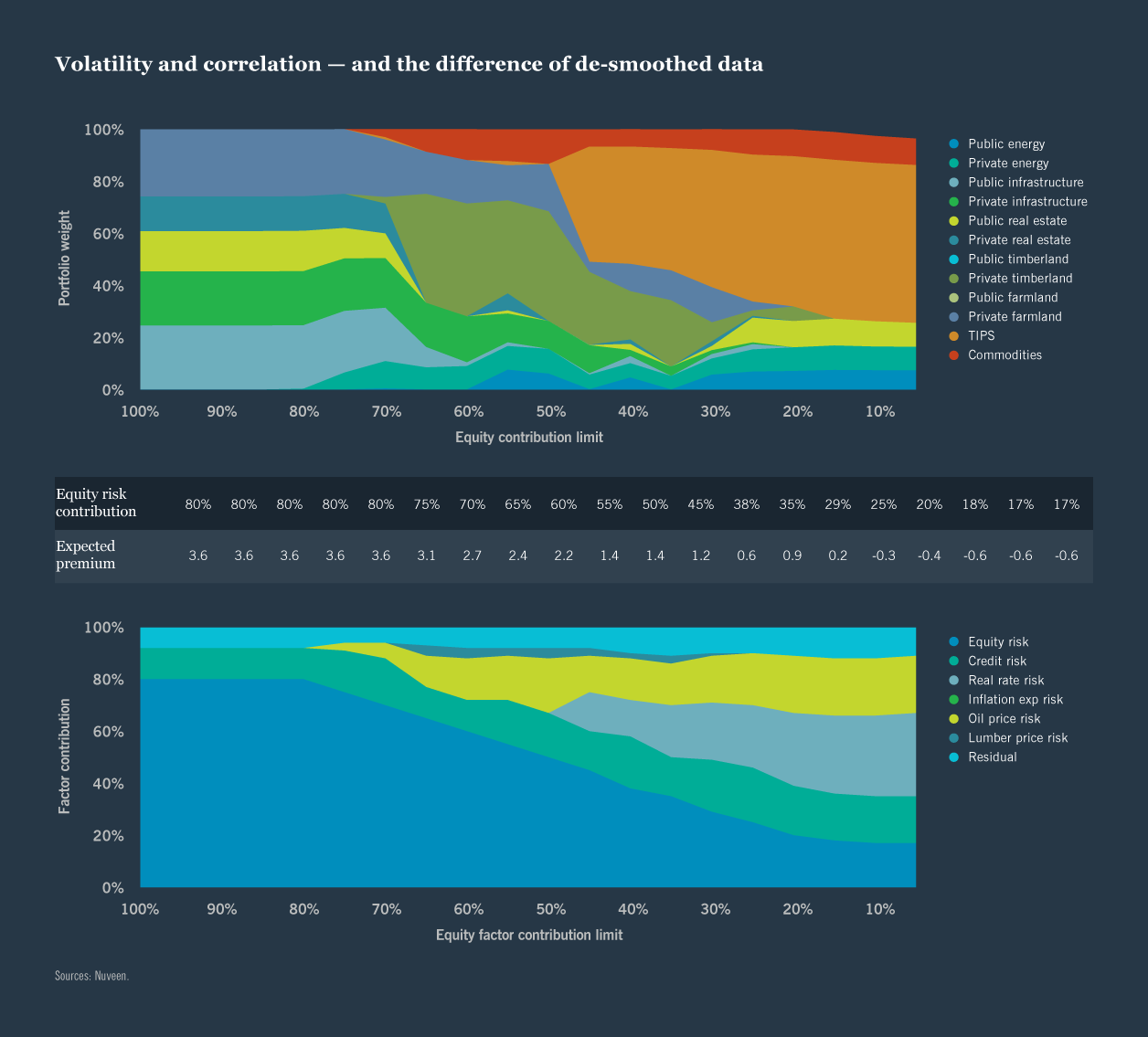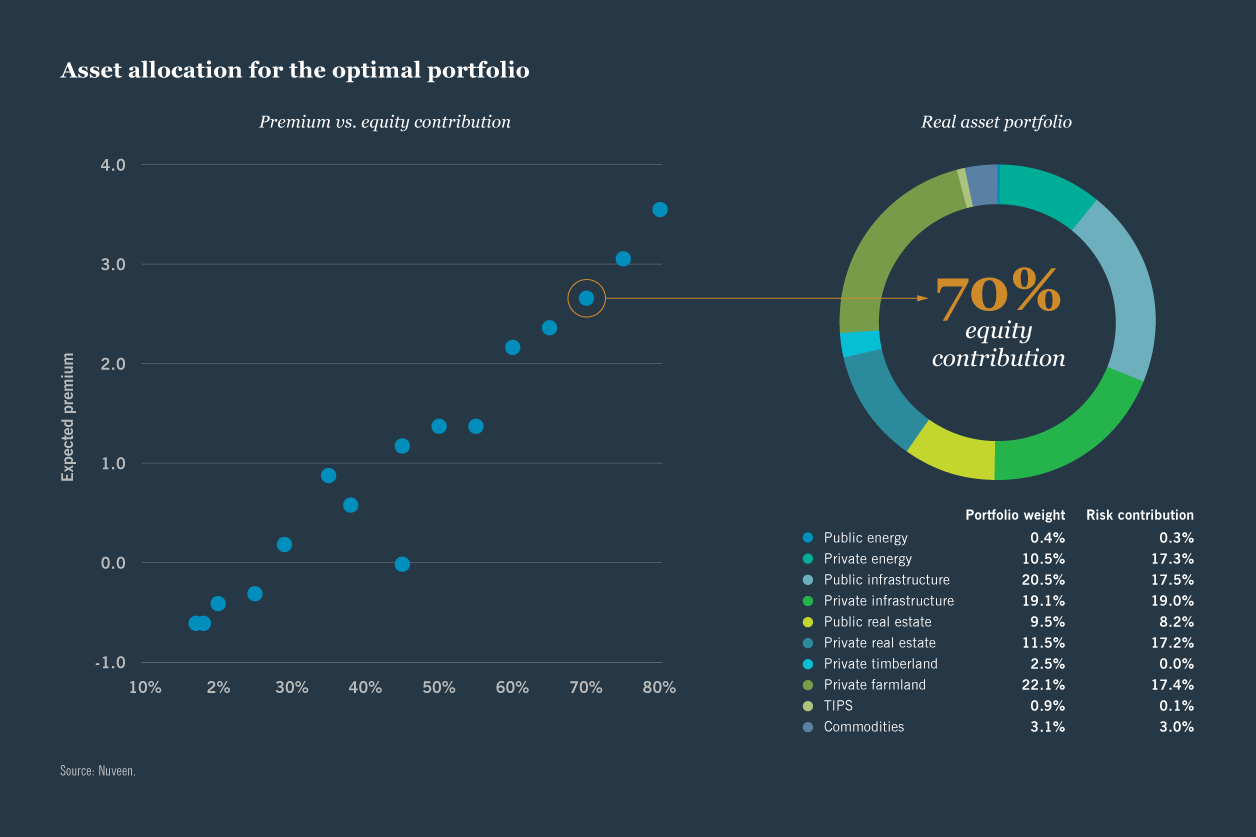TOOLS
Real assets

# A portfolio construction case study## Portfolio construction with real asset factors

Factor analysis lays the foundations for building a real asset portfolio that meets specific investment objectives.

Building on the factor analysis work in the preceding section, we illustrate how a public pension plan can use this work to construct a portfolio with explicit factor exposure targets. This approach can help maximize the diversification benefits of the real asset portfolio. Furthermore, it can be customized to meet the needs of a range of institutional plans with their own unique objectives and circumstances.

In this example, the investor is a public pension plan with significant exposure to equity risk. Given this, the objective is to construct a real assets portfolio that brings real diversification to the overall portfolio. To do so, the portfolio construction process aims to maximize two elements:
1. The diversification benefits of a custom real asset portfolio
2. The asset premiums beyond those that can be accessed via common factors

To balance these potentially competing objectives, we introduce the following constraints: long-only investments, 20% risk budget limit from any one sub-asset class, and variable equity risk contribution limits to develop a frontier of portfolios that maximize non-common factor premiums and limit equity risk exposure. Also in this example, the investor has no binding liquidity constraint. We recognize that many other institutions will have liquidity requirements. A liquidity constraint can be added to the framework by limiting the amount of private vs. public real asset exposure in the portfolio.

This framework can be used by any institutional investor seeking to target other risk factors. For example, well-funded corporate pension plans following a de-risking strategy may be particularly focused on interest rate risk. The framework is flexible enough to incorporate the macro factor risk most relevant to the institution and also work with different constraints regarding types of investment, risk budgets and liquidity needs.

### Initial data requirements and analysis

We begin with the following information:
1. The institution’s current portfolio: This is a full breakdown of the assets and the proportion in which they are held in the entire portfolio, required for the factor analysis.
2. The opportunity set of real asset strategies for consideration: This may be the full range of public and private strategies, but it can exclude strategies unsuited to the investor, perhaps due to lack of alignment with investment philosophy or for size. Factor exposures will be estimated for all applicable strategies.
3. The expected asset-specific and illiquidity return premiums: These can be provided by our analysis or the investor may wish to incorporate their own premium estimates.
For this exercise, the institution’s current portfolio is based on the average asset allocation weights for state and local pension plans in the United States displayed in the figure below. Using the analysis techniques illustrated in the prior section, the figure below illustrates the contribution to total risk coming from each of the factors analyzed. Over 99% of the risk is from exposure to the common factors, and the vast majority of the risk in the average public pension plan’s portfolio is from equity risk exposure. We use a hypothetical set of premiums that incorporate negative views on energy and positive views on the other real asset classes, illustrated in the table below.Over 99% of the risk is from exposure to the common factors, and the vast majority of the risk in the average public pension plan’s portfolio is from equity risk exposure.

### Optimizing the portfolio

Using the inputs developed in the preceding sections, we develop a frontier of optimal real asset portfolios at various equity contribution limits and premiums, illustrated in the top chart of the figure below.We see that as we limit the equity factor contribution, different real assets play a role in the portfolio, highlighting their diversification qualities. In this case, allocations to timberland and inflation-linked U.S. Treasury bonds (TIPs) increase substantially with the reduction in equity risk factor contribution. Of course, as we see from the table, limiting equity risk impacts the expected premium. It declines.

We also see an increase in other risks in the lower chart. It shows the changing factor risk profile for the different allocations. In this example, there is a notable increase in oil risk and real rate risk. A key decision for the investor is to determine their willingness to trade equity risk for other risk factors.

For illustrative purposes, we selected the real asset portfolio in the figure below. It limits the equity risk contribution to 70% and distributes the residual risk across a wide range of sub asset classes. Infrastructure is the largest exposure, split between public and private investments. Real estate exposure is second, again split between public and private. Public investments comprise over a third of the portfolio. Private farmland investments are the largest single exposure.Residual risk is spread across a wide range of sub asset classes.

This exercise is based on sample inputs to illustrate the portfolio construction process. However, the framework can be customized with the investor’s own return premium expectations, specific investment constraints and existing portfolio composition.

## Continue reading: Think real assets

Real assets

Real assets

Real assets

#### The factors driving real asset returns